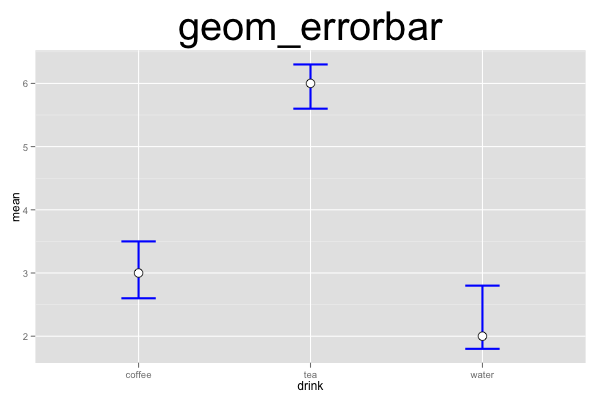## ggplot2 Quick Reference: geom_errorbar

A geom that draws error bars, defined by an upper and lower value. This is useful e.g., to draw confidence intervals.

An error bar is similar to a pointrange (minus the point, plus the whisker). It is also similar to a linerange (plus the whiskers).

Default statistic: stat_identity

### Parameters

• x - (required) x coordinate of the bar
• ymin - (required) y coordinate of the lower whisker
• ymax - (required) y coordinate of the upper whisker
• size - (default: 0.5) thickness of the lines
• linetype - (default: 1=solid) the type of the lines
• colour - (default: "black") the color of the lines
• width - (default: 0.9) width of the whiskers
• alpha - (default: 1=opaque) the transparency of the lines

### Example

This plot consists of two layers. The bottom layer shows error bars, and the top layer shows points. Note that we have to provide (or compute) the ymin and ymax values for the error bars ourselves (the errorbar geom does not automatically compute a confidence interval).```d=data.frame(drink=c("coffee","tea","water"), mean=c(3,6,2), lower=c(2.6,5.6,1.8), upper=c(3.5,6.3,2.8))
ggplot() +
geom_errorbar(data=d, mapping=aes(x=drink, ymin=upper, ymax=lower), width=0.2, size=1, color="blue") +
geom_point(data=d, mapping=aes(x=drink, y=mean), size=4, shape=21, fill="white") +
opts(title="geom_errorbar", plot.title=theme_text(size=40, vjust=1.5))```